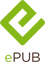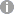# Wavelet Analysis and Transient Signal Processing Applications for Power Systems

Wiley (Verlag)
• 1. Auflage
• |
• erschienen am 12. April 2016
• |
• 264 Seiten

 E-Book | ePUB mit Adobe DRM | Systemvoraussetzungen
978-1-118-97703-3 (ISBN)

An original reference applying wavelet analysis to power systems engineering
* Introduces a modern signal processing method called wavelet analysis, and more importantly, its applications to power system fault detection and protection
* Concentrates on its application to the power system, offering great potential for fault detection and protection
* Presents applications, examples, and case studies, together with the latest research findings
* Provides a combination of the author's tutorial notes from electrical engineering courses together with his own original research work, of interest to both industry and academia
 Reihe: Sprache: Englisch Verlagsort: Hoboken | Singapur Verlagsgruppe: John Wiley & Sons Dateigröße: 27,66 MB Schlagworte: ISBN-13: 978-1-118-97703-3 (9781118977033) ISBN-10: 1-118-97703-3 (1118977033)weitere Ausgaben werden ermittelt
Preface
Chapter 1: Introduction
Chapter 2: The Fundamental Theory of Wavelet Transform
Chapter 3: Wavelet analysis and signal singularity
Chapter 4: The sampling technique in the wavelet analysis of transient signals
Chapter 5: Selection of wavelet basis for transient signal analysis of power system
Chapter 6: Construction Method of Practical Wavelet in Power System Transient Signal Analysis
Chapter 7: Wavelet post-analysis methods for transient signals in power system
Chapter 8: Application of wavelet analysis in high-voltage transmission line fault location
Chapter 9: Application of wavelet transform in fault feeder identification in the neutral ineffectively grounded power system
Chapter 10: Application of wavelet transform to non-unit transient protection
Chapter 11: The application of wavelet analysis to power quality disturbances
Chapter 12: Wavelet entropy definition and its application in power system transient signals detection and identification
Appendix A: Simulation Models
Index

# 1Introduction

## 1.1 From Fourier transform to wavelet transform

### 1.1.1 Fourier transform 

Information in the time domain and frequency domain is the basic characteristic of the description of a signal x(t). Information in the time domain is easy to observe, whereas information in the frequency domain is not observable unless the signal transforms. Fourier first proposed the method to get frequency domain information when he was researching an equation of heat conduction. Moreover, he suggested transforming the equation of heat conduction from the time domain to the frequency domain, which is the famous Fourier transform concept. The definition of Fourier transform of a continuous signal x(t) is

(1.1)

Fourier transform established the relation between the time domain and frequency domain of a signal.

With the development of computer technology, all the computing problems in science and engineering now relate to computers inextricably. A typical feature of computer computing is discretization. Fourier transform, defined in Equation (1.1), is essentially integral computation, which reflects continuous characteristics. Meanwhile, discrete sampling in applications obtains signals. Equation (1.1) needs to be discretized with high efficiency and high accuracy in order to sample information through discretization and compute Fourier transform effectively using computers. Thus, one must derive the definition of discrete Fourier transform (DFT).

Let x(t) be a limited signal over the interval [-p,p], so Fourier transform of x(t) can be simplified as

(1.2)

Moreover, let the signal be equidistant sampled. The sampling number is N, the input signal in the time domain is xk, and the output signal required in the frequency domain is Xk. To get more accurate output Xk of Equation (1.1) using sampling points of input xk, DFT is a polynomial of best approximation S(t) fitting from x(t), according to xk, and having S(t) instead of x(t) in Equation (1.1) to get Xk. The following paragraph briefly discusses the solution of S(t) and Xk.

Using a given group of orthogonal basis () to verify the inner product relations of the vectors: , in which IN is an identity matrix for N dimensions, and .

Let , and use orthogonal basis {Fk}, to solve the least-square problem:

(1.3)

Solution of Equation (1.3):

(1.4)

Use definition of S(t) and coefficient ck from Equation (1.4) to approach Xk.

Its DFT is a translation by coefficient ck of the polynomial S(t) of x(t):

(1.5)

Except for 2p, DFT is defined by Equation (1.5), where the input xn and output Xl are the time domain information and frequency domain information of the signal, respectively.

### 1.1.2 Short-time Fourier transform [1, 2]

Although Fourier transform and DFT have been applied to signal processing, especially time-frequency analysis, extensively, the Fourier integral cannot be localized in both the time and frequency neighborhoods at one time. For example, according to Equation (1.1), Fourier transform cannot analyze the time evolution of such spectral components and the signal cycle. That is to say, Fourier transform cannot localize in the frequency neighborhoods. Actually, the signal is transformed from the time domain to frequency domain by Fourier transform, where was added at all points in time and was the limitation of frequency. Thus, Fourier transform is not a survey of frequency domain information of a signal in a period. In contrast, for signal processing, especially nonstationary signal processing (voice, seismic signal, etc.), local frequency of signal and its period should be realized. Because standard Fourier transform has the ability of local analysis in the frequency domain rather than time domain, Dennis Gabor proposed short-time Fourier transform (STFT) in 1946. The basic idea behind STFT is dividing the signal into many time intervals to analyze each of them and determine their frequencies. Figure 1.1 is a sketch of signal analysis using Fourier transform.

Figure 1.1 Diagram of short-time Fourier transform: (a) window in time domain, (b) time-frequency plane division

We assume that we are only interested in the frequency of x(t) neighboring t = t, which is the value of Equation (1.1) in a certain period It. We have

(1.6)

With |It| representing the length of the period It, we definite the square wave function gt(t) as

(1.7)

So Equation (1.6) can be written as

(1.8)

with i representing the whole real axis. According to Equations (1.1), (1.7), and (1.8), to analyze the local frequency domain information at time t, Equation (1.6) is the windowed function gt(t) of function x(t). Obviously, the smaller the length |It| of the window is, the more easily the function could reflect the local frequency domain information of signals.

The definition of STFT is given in Reference .

For a given signal , STFT can be written as

(1.9)

with

(1.10)

and

The windowed function g(t) should be symmetrical. The meaning of STFT is as follows. Add a window function g(t) to x(t). The time variable of x(t) and g(t) changes from t to t. The windowed signal transforms to get the Fourier transform of the signal at time t. A moving time t, which means a moving central position of the windowed function, can derive the Fourier transform at different times. The set of these Fourier transforms is STFTx(t, ?), as shown in Figure 1.2. STFTx(t, ?) is a two-dimensional function of variables (t, ?).

Figure 1.2 Diagram of STFT

The windowed function g(t) is finite supported in the time domain, and ei?t is a line spectrum in the frequency domain. Thus, the basis function of STFT is finite supported in both the time and frequency domains. In this way, the inner product of Equation (1.9) can realize the function of time-frequency locating for x(t). Fourier transform on both sides of Equation (1.10):

(1.11)

in which ? is the equivalent frequency variable the same as ?.

(1.12)

Thus,

(1.13)

The equation indicates that windowed x(t) in the time domain (which is ) is equal to windowed X(?) in the frequency domain (which is ).

STFT solved the problem that standard Fourier transform has the ability of local analysis only in the frequency domain rather than the time domain. However, STFT is itself flawed because a fixed windowed function whose form and shape don't change determine the resolution of STFT. To change the resolution is to choose a different windowed function. STFT is effective when you use it to analyze segment-wise stationary signals or approximate-stationary signals. But nonstationary signals, which change dramatically, demand high time resolution. When the signal is relatively flat, such as a low-frequency signal, it demands a windowed function with high-frequency resolution. STFT cannot balance the demand of both frequency resolution and time resolution.

### 1.1.3 Time-frequency analysis and wavelet transform

In view of the analysis in Section 1.1.2, Fourier transform can reveal a signal's frequency domain feature and the energy feature of a stationary signal. That is to say, Fourier transform is an overall transformation of signal, which is completely in the time domain or frequency domain. Therefore, it cannot reveal the law of the time-varying signal spectrum. For a nonstationary signal, because of large variations in a spectrum, analysis method is demanded to reflect local time-varying spectrum features of signal instead of simply those in the time domain or frequency domain. To make up the shortcoming of Fourier transform, a novel method that can realize time-frequency localization for signals is essential. Consequently, the time-frequency analysis was proposed, which can represent signals in the time and frequency domain at the same time.

During the development of Fourier transform theory, people realized its shortcomings (mentioned in this chapter). Therefore, in 1946, Gabor proposed representing one signal with both a time axis and frequency axis. The Gabor expansion of signal x(t) is [3-5]:

(1.14)

The windowed function...

Schweitzer Klassifikation
Thema Klassifikation
DNB DDC Sachgruppen
Dewey Decimal Classfication (DDC)
• Technology
•Engineering
•Applied physics
BISAC Classifikation
• Science
•Energy
Warengruppensystematik 2.0Dateiformat: EPUB
Kopierschutz: Adobe-DRM (Digital Rights Management)

Systemvoraussetzungen:

Computer (Windows; MacOS X; Linux): Installieren Sie bereits vor dem Download die kostenlose Software Adobe Digital Editions (siehe E-Book Hilfe).

Tablet/Smartphone (Android; iOS): Installieren Sie bereits vor dem Download die kostenlose App Adobe Digital Editions (siehe E-Book Hilfe).

E-Book-Reader: Bookeen, Kobo, Pocketbook, Sony, Tolino u.v.a.m. (nicht Kindle)

Das Dateiformat EPUB ist sehr gut für Romane und Sachbücher geeignet - also für "fließenden" Text ohne komplexes Layout. Bei E-Readern oder Smartphones passt sich der Zeilen- und Seitenumbruch automatisch den kleinen Displays an. Mit Adobe-DRM wird hier ein "harter" Kopierschutz verwendet. Wenn die notwendigen Voraussetzungen nicht vorliegen, können Sie das E-Book leider nicht öffnen. Daher müssen Sie bereits vor dem Download Ihre Lese-Hardware vorbereiten.

Weitere Informationen finden Sie in unserer E-Book Hilfe.